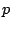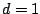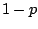Next: One-pole, one-zero high-pass filter Up: Designing filters Previous: Designing filters   Contents   Index

## One-pole low-pass filterThe one-pole low-pass filter has a single pole located at a positive real number, as pictured in Figure 8.12. This is just a recirculating comb filter with delay length, and the analysis of Section 7.4 applies. The maximum gain occurs at a frequency of zero, corresponding to the point on the circle closest to the point. The gain there is. Assumingis close to one, if we move a distance ofunits up or down from the real (horizontal) axis, the distance increases by a factor of about, and so we expect the half-power point to occur at an angular frequency of about.

This calculation is often made in reverse: if we wish the half-power point to lie at a given angular frequency, we set. This approximation only works well if the value ofis well under, as it often is in practice. It is customary to normalize the one-pole low-pass filter, multiplying it by the constant factorin order to give a gain of 1 at zero frequency; nonzero frequencies will then get a gain less than one.

The frequency response is graphed in Figure 8.12 (part b). The audible frequencies only reach to the middle of the graph; the right-hand side of the frequency response curve all lies above the Nyquist frequency.

The one-pole low-pass filter is often used to seek trends in noisy signals. For instance, if you use a physical controller and only care about changes on the order of 1/10 second or so, you can smooth the values with a low-pass filter whose half-power point is 20 or 30 cycles per second.Next: One-pole, one-zero high-pass filter Up: Designing filters Previous: Designing filters   Contents   Index
Miller Puckette 2006-12-30